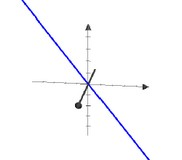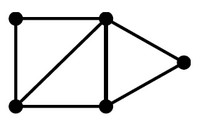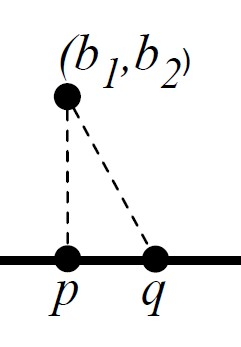# Linear Algebra - Span of a Vector Space

The set of all linear combinations of some vectors v1,…,vn is called the span of these vectors and contains always the origin.

Example: Let V = Span {[0, 0, 1], [2, 0, 1], [4, 1, 2]}. A vector belongs to V when you can write it as a linear combination of the generators of V.

Related to Graph - Spanning ?

## Definition

The set of all linear combinations of some vectors v1,…,vn is called the span of these vectors and contains always the origin.## How to

### know if a vector is in the span

How to know if a vector is in the Span {v1, . . . , vn} ?

Let $A = \begin{bmatrix} \begin{array}{r|r|r} && \\ v_1 & \dots & v_n \\ && \end{array} \end{bmatrix}$

Using the linear-combinations interpretation of matrix-vector multiplication, a vector x in Span {v1, . . . , vn} can be written Ax.

Thus testing if b is in Span {v1, . . . , vn} is equivalent to testing if the matrix equation Ax = b has a solution.

## Example

### Span {}

The Span {} have only one vector: the zero vector

### Span {[1, 1], [0, 1]} over gf2

The Span {[1, 1], [0, 1]} over the field GF(2) is composed of the following linear combinations:

0 [1, 1] + 0 [0, 1] = [0, 0]
0 [1, 1] + 1 [0, 1] = [0, 1]
1 [1, 1] + 0 [0, 1] = [1, 1]
1 [1, 1] + 1 [0, 1] = [1, 0]


Thus there are four vectors in the span.

### Span {[2, 3]} over $\mathbb{R}$

The Span {[2, 3]} over $\mathbb{R}$ contains an infinite number of vectors. They forms the line through the origin and (2, 3).### Span of two vectors

The span of two vectors is a plane containing the origin.

### Span in another Span

[3, 0, 0], [0, 2, 0], and [0, 0, 1] are in Span {[1, 0, 0], [1, 1, 0], [1, 1, 1]}:

[3, 0, 0] = 3 [1, 0, 0]
[0, 2, 0] = 2 [1, 0, 0] + 2 [1, 1, 0]
[0, 0, 1] = - 1 [1, 0, 0] - 1 [1, 1, 0] + 1 [1, 1, 1]


## Dimension

The span of k vectors is not always k-dimensional

• Span {[0, 0]} is 0-dimensional.
• Span {[1, 3], [2, 6]} is 1-dimensional as [1, 3] = 1/2 x [2, 6]
• Span {[1, 0, 0], [0, 1, 0], [1, 1, 0]} is 2-dimensional as [1, 0, 0] + [0, 1, 0] = [1, 1, 0]

To predict the dimensionality of the span of some vectors, compute the rank of the set of vectors.

## Exchange Lemma

Suppose S is a set of vectors and A is a subset of S. Suppose z is a vector in Span S such that $A \cup \{z\}$ is linearly independent. Then there is a vector $w \in S − A$ such that: $$Span S = Span (S \cup \{z\} − \{w\})$$

Discover MoreGeometry - Object

in Geometry An object can be: a pre-defined shape or an arbitrary one defined with the help of a path. Notions such as: The length of a vector The angle between two vectors. are introduced...Graph - Spanning

A set S of edges is spanning for a graph G if, for every edge {x, y} of G, there is an x-to-y path consisting of edges of S. ie for each edge xy in G, there is an x-to-y path consisting of edges of S....Linear Algebra - Basis of a Vector Space

A basis for vector space V is a linearly independent set of generators for V. Thus a set S of vectors of V is a basis for V if S satisfies two properties: Property B1 (Spanning) Span S = V, and Property...Linear Algebra - Closest Point on a Line

How to find the closest point on a line from a point ? How to find the vector on the line that best approximates the given vector b (the closest point on the line) Letb be a vector Leta be a...Linear Algebra - Closest point in higher dimension than a plane

Solving closest point in the span of many vectors Goal: An algorithm that, given a vector b and vectors v1, . . . , vn, finds the vector in Span {v1, . . . , vn} that is closest to b. Special case:...Linear Algebra - Find a basis computation problem

Find a basis for a vector space Example: Find a basis for the null space of By the dot-product definition of matrix-vector multiplication, a vector v is in the null space of A if the dot-product...Linear Algebra - Linear Dependency

Vectors v1, . . . , vn are linearly dependent if the zero vector can be written as a nontrivial linear combination of the vectors: In this case, we refer to the linear combination as a linear dependency...Linear Algebra - Matrix

The Traditional notion of a matrix is: a two-dimensional array a rectangular table of known or unknown numbers One simple role for a matrix: packing together a bunch of columns or rows Matrix...Linear Algebra - Orthogonalization - Building an orthogonal set of generators

Original stated goal: Find the projection of b orthogonal to the space V spanned by arbitrary vectors Input: A list of vectors over the reals output: A list of mutually orthogonal vectors such...Linear Algebra - Rank

in linear algebra The rank of a set S of vectors is the dimension of Span S written: rank S dim Any set of D-vectors has rank at most |D|. If rank(S) = len(S) then the vectors are linearly dependent...